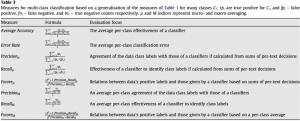# What are evaluation metrics for multi-class classification problem (like positive/negative/neutral sentiment analysis)

For multiclass classification(MCC) problems, metrics  can be derived from the confusion matrix. Let $tp_i,tn_i,fp_i,fn_i$ denote the true positives, true negatives, false positives, false negatives respectively.

MCC problems, usually macro and micro metrics are computed:

→ Micro metrics (with subscript $\mu$ in table below) are computed by summing up individual tp, tn, fp and fn to extend the two class formula for precision and recall for MCC.

→ Macro metrics are computed  by taking the average precision, recall of the system on different sets treating classifier for each class as a 1 vs all classifier.

See the table below for popular micro and macro metrics for multi class classification.